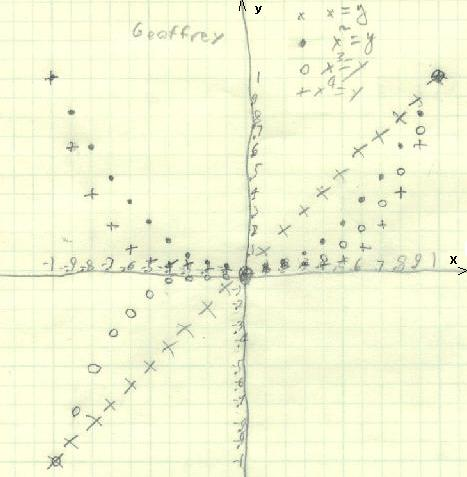Geoffrey, going into 5th grade, graphed x = y,  x2 = y,  x3 = y, and  x4 = y from x = -1 to 1 by 0.1's

Geoffrey had figured out the rule for the shuttle puzzle P(P+2)=M, and Don asked him to graph this function as x(x+2)=y. He got a parabola. They looked at the pattern in the parabola- from (0,0) it goes 1 right, 1 up, 1 right then 3 up, 1 right then 5 up, and continued going up the odd numbers. Don then asked Geoffrey to graph x = y,  x2 = y,  x3 = y, and  x4 = y from x = -1 to 1 by .1's, on the same graph paper. He did this below:Then Don asked him to write about what he found in the graphs.

"When x is negative and y = x raised to an odd power, both x and y are negative because if you multiply -.9 by -.9 by -.9 you have to get a negative number because a negative times a negative is a positive , and a negative times a positive is a negative.

When x4 =y the graph looks more like half a square because when you multiply a number between 0 and 1 and you make it to the fourth power it gets much smaller because it's kind of like dividing because .12=.1 x .1=.01".

Geoffrey worked on moving the parabola y=x2 up 2 units (he found this equation to be y= x2 +2) and to the right 3 units (he found this equation to be y= (x - 3)).

Fine job Geoffrey!

Geoffrey has been working on the SSAT test off and on, getting ready for the test next year. Don showed Geoffrey how to multiply 12x13 in his head, and ended up multiplying 22x23 in his head. Don also showed geoffrey how to square 2 numbers the end in 5, like 25x25 =  ?  Well the answer has a 25 on the right  _ 2 5 . Take the other number 2, add 1, to get 3, then multiply by 2 by 3 to get 6. The answer to 25x25 = 625. In the process of doing something in school he talked about 58 = 6252 and he proceeded to multiply 625x625 in his head!

To Geoffrey's work on powers of powers
To Geoffrey's work on the area under curves and the integral
To Geoffrey's work on infinite series
To Geoffrey's work on the Fibonacci numbers
To Geoffrey's work on the 6 trig functions
To other discoveries
To order Don's materials
Mathman home